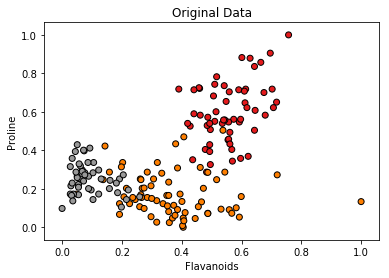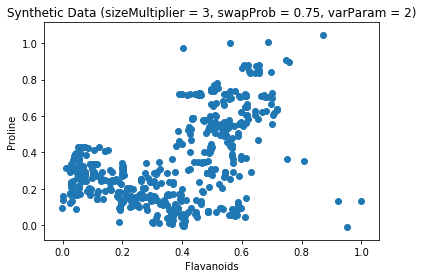# Model Compression and MUNGE

In this post, I will summarize the paper titled Model Compression , and implement the synthetic data generation algorithm described in it.

We know that ensembles perform well, but their computational cost is too high. This paper proposes compressing an ensemble into a lightweight model by labeling an unlabeled dataset with the ensemble, and training the lightweight model on the newly labeled dataset. By doing that, the lightweight model mimics the knowledge of the ensemble. Also, the lightweight model trained on the newly labeled dataset outperforms the lightweight model trained on the original dataset. This result suggests that we are able to transfer the knowledge in the ensemble to the lightweight model.

If there is no unlabeled data to label with the ensemble, one can choose to generate synthetic data. However, care needs to be taken, because synthetic data should match the distribution of the original data. The authors propose MUNGE, an algorithm for data generation. I could not find a Python implementation and implemented it myself.

``````def findNearestNeighbor( elementIndex, array):
"""
elementIndex: the element that we are searching the nearest neighbor of
array: array

Returns
-------
nearestNeighborIndex: the index of the nearestNeighbor
"""
element = array[elementIndex]
array = np.delete( array, elementIndex, axis=0) # prevent elementIndex == nearestNeighborIndex
nearestNeighborIndex = np.argmin( np.linalg.norm( element - array, axis=1))

if nearestNeighborIndex >= elementIndex: # adjust
nearestNeighborIndex += 1
return nearestNeighborIndex

def munge( dataset, sizeMultiplier, swapProb, varParam):
"""
This algorithm was presented in the following paper:

C. Bucilua, R. Caruana, and A. Niculescu-Mizil. Model compression. In Proceedings of the
12th ACMSIGKDD International Conference on Knowledge Discovery and Data Mining, KDD
’06, pages 535–541, New York, NY, USA, 2006. ACM.

Creates a synthetic dataset.
Continuous attributes should be linearly scaled to [0, 1].
For now, assumes all attributes are continuous and the dataset is 2D. #TODO

dataset: (numExamples, numAttributes)
sizeMultiplier: dataset size multiplier
swapProb: probability of swapping attributes (draw from normal with mean)
varParam: local variance parameter

Returns
-------
synthetic: (sizeMultiplier*numExamples, numAttributes)
"""

numExamples, numAttributes = dataset.shape
synthetic = np.empty((sizeMultiplier*numExamples, numAttributes))

for i in range( sizeMultiplier):
tempDataset = np.copy(dataset)

for exampleIndex in range( numExamples):
nearestNeighborIndex = findNearestNeighbor( exampleIndex, tempDataset)

for j in range( numAttributes):
if np.random.uniform() < swapProb:
example_attr = tempDataset[ exampleIndex, j]
closestNeighbor_attr = tempDataset[ nearestNeighborIndex, j]

tempDataset[ exampleIndex, j] = np.random.normal( closestNeighbor_attr, abs( example_attr - closestNeighbor_attr) / varParam)
tempDataset[ nearestNeighborIndex, j] = np.random.normal( example_attr, abs( example_attr - closestNeighbor_attr) / varParam)

synthetic[i*numExamples:(i+1)*numExamples, :] = tempDataset
return synthetic
``````

For each example `e` in the dataset, we find its nearest neighbor `e'`. Then, with probability `swapProb`, each attribute `e_a` is assigned a random value drawn from normal distribution with mean `e_a'` and standard deviation `|e_a - e_a'|/varParam`, and vice versa (`e_a'` is assigned a random value from normal with mean `e_a`). An experiment with the sklearn Wine Recognition Dataset can be seen below.It is important to note that the synthetic data is very sensitive to `swapProb` and `varParam`. Although MUNGE is simple, variational autoencoders or GANs (GANs are the state-of-the-art at the moment) should be used for data generation.

The source code of the experiment can be found here.

 C. Bucilua, R. Caruana, and A. Niculescu-Mizil. Model compression. In Proceedings of the 12th ACMSIGKDD International Conference on Knowledge Discovery and Data Mining, KDD’06, pages 535–541, New York, NY, USA, 2006. ACM.